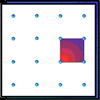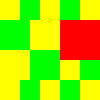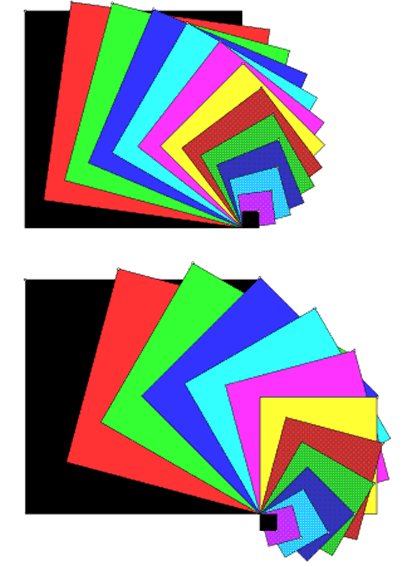#### You may also like### Geoboards

This practical challenge invites you to investigate the different squares you can make on a square geoboard or pegboard.### Tiles on a Patio

How many ways can you find of tiling the square patio, using square tiles of different sizes?### Pebbles

Place four pebbles on the sand in the form of a square. Keep adding as few pebbles as necessary to double the area. How many extra pebbles are added each time?

# Overlapping Squares

##### Age 7 to 11Challenge LevelDescribe what is happening in these pictures.

Do the pictures appear to be coming towards you, or going away?

How many squares are there in each picture?

If the side of the smallest square is 1 unit, how many units is the side of the next square?
And the next?
How many units is the side of the largest square?

What is the area of the smallest square?
What is the area of the next square? And the next?
What is the sequence of the areas of the squares?

The smallest square is one unit of area. How many more units are in the second square?
How many more units of area are in the third square than in the second square?
What is the rule for building the squares?

Look at the top picture. Start from the largest square and think of it as shrinking as it turns.
Through what angle has it turned when it has changed to the smallest square?
Is it the same in both pictures?
What is the angle of rotation as one square changes to the next?
Is it the same in both pictures?

Make a similar picture. You could change the rules if you would like to.